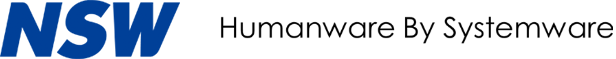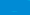#### Financial Highlights

• Net sales

(Millions of yen)IT solutionsProduct solutions
• Operating income/
Operating income ratio
(Millions of yen)
(%)Operating incomeOperating income ratio
• Ordinary income/
Ordinary income ratio
(Millions of yen)
(%)Ordinary incomeOrdinary income ratio
• Net income/Net income ratio
(Millions of yen)
(%)Net incomeNet income ratio
• Total assets/ROA
(Millions of yen)
(%)Total assetsReturn on assets[ROA]
• Total equity/ROE
(Millions of yen)
(%)Total equityReturn on equity[ROE]
(Millions of yen)

Net sales
IT solutions
Product solutions
Operating income
Operating income ratio(%)
Ordinary income
Ordinary income ratio(%)
Net income
Net income ratio(%)
Total assets
Return on assets[ROA](%)(*1)
Total equity(*2)
Return on equity[ROE](%)(*3)

(*1)Return on assets (ROA) = Ordinary income ÷ Total assets during the period × 100 (%)

(*2)Total equity = Total assets - Minority interests

(*3)Return on equity (ROE) = Net income ÷ Total equity during the period × 100 (%)